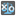• # How to refactor to not use cascading returns?

## Question related to missionXs and Os Referee

This has been a problem I've had in other projects, and I'm wondering if anyone can give me feedback on how to design/refactor this code so that it doesn't rely on a structure like this?

```def checkio(game_result):
winner = verticalWinner(game_result)
if (winner != "."):
return winner

winner = horizontalWinner(game_result)
if (winner != "."):
return winner

winner = diagonalWinner(game_result)
if (winner != "."):
return winner

return "D"

def horizontalWinner(game_result):
for row in game_result:
if (row == row == row != '.'):
return row
return "."

def verticalWinner(game_result):
for i in range(3):
if (game_result[i] == game_result[i] == game_result[i] != '.'):
return game_result[i]
return "."

def diagonalWinner(game_result):
winner = diagonalSW(game_result)
if (winner != "."):
return winner

winner = diagonalNW(game_result)
if (winner != "."):
return winner

return "."

def diagonalSW(game_result):
if (game_result == game_result == game_result != '.'):
return game_result
return "."

def diagonalNW(game_result):
if (game_result == game_result == game_result != '.'):
return game_result
return "."
```12